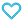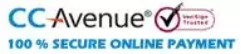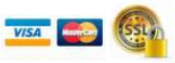# Class 3-Integrated Learning Mathematics with NEP Guidelines

595.00

• Paper Book

MTG Learning Mathematics for a Smarter Life textbook provides learners and teachers with a one-of-a-kind learning experience. The book strictly follows NEP 2020 guidelines and focuses on experiential learning rather than rote learning. The book contains simple text with visuals and illustrations, followed by a series of sections such as word fun, yeah, I can do it now, miscellaneous practice, and wrap-up to help learners improve their problem-solving skills. Activities and IMO questions at the end make the book a complete learning package that improves the learners’ critical thinking and creativity.

881 in stock

Qty:Add to WishlistMTG’s Learning Mathematics for a Smarter Life is a creative Mathematics series for students in grades 1 through 8. The goal of this series is to encourage students to enjoy their studies and learn concepts deeply rather than rote learning as per the NEP 2020 guidelines. The series is designed with the student’s age groups in mind, so they can learn at their own pace. Higher Order Thinking Skills (HOTS) and SOF International Mathematics Olympiad (IMO) questions are included in the series to help students improve their analytical and problem-solving skills. The following key features of the books contribute to a unique learning experience for both children and teachers. The section names are also chosen with care so that students can identify with them.

• “Brief Introduction”- gives brief information and explains each topic step by step with illustrative examples.
• “Getting Ready “connects the chapter with lessons learned previously.
• “Do it yourself” – after completing each topic, this unique section helps in building the confidence level among the students.
• “Practice Work” helps the students to brush up on their application skills.
• “Wrap Up” helps in recapitulates the entire chapter in a creative manner.
• “Teacher’s Note” is given as suggestions and tip notes for teachers to make their teaching more effective and convenient.
• “Word Fun” helps in building connections of mathematics with day-to-day life.
• “Activities” is to develop the observation, concentration, and analyzing power among the students.
Table of Content:
• 1. Number System
• Numbers Beyond 999
• Numbers Beyond 1000
• Place Value & Face Value
• Numbers on Abacus &
• Number Name
• Expanded Form and Short Form
• Comparison of Numbers
• Forming Number (Without Repetition)
• Rounding Off Numbers
• More Than and Less Than
• Even and Odd Numbers
• Roman Numerals
• Wrap Up
• Miscellaneous Practice
• (Without Regrouping)
• Regrouping
• Addition of four digit Numbers
• (With Regrouping)
• Adding : 10, 100, 1000 and 99, 999
• Estimating the Sum
• Word Fun
• Wrap Up
• Miscellaneous Practice
• 3. Subtraction
• Subtraction of 4-digit Numbers
• (Without Regrouping)
• Regrouping with Zero
• Subtraction of 4-digit Numbers
• (With Regrouping)
• Subtraction Properties
• Checking Subtraction
• Estimating the Difference
• Word Fun
• Wrap Up
• Miscellaneous Practice
• 4. Multiplication
• Multiplication Tables
• Factors and Product
• Word Fun
• Multiplication Properties
• Multiplication by 10, 20, ….., 90 and
• 100, 200, ….., 900
• Multiplication of a 2, 3 or
• 4-Digit Number by a 1-Digit Number
• (Without Regrouping)
• Multiplication of a 2, 3, 4-Digit
• Number by a 1-Digit Number
• (With Regrouping)
• Multiplication of a 2 or 3-Digit Number
• by a 2-digit Number and Multiplication
• of Three 1-digit Numbers
• Word Fun
• Wrap Up
• Miscellaneous Practice
• 5. Lines and Shapes
• Simple Concepts in Geometry
• Solids (3D-Shapes)
• Faces, Edges and Vertices in Solid Objects
• Open and Closed Figures
• Figures having Same Shape and Same Size
• Wrap Up
• Miscellaneous Practice
• 6. Patterns and Symmetry
• Patterns and Rangoli Pattern
• Tiling Pattern
• Number Patterns
• Figure Patterns
• Exploring Patterns
• Top View and Side View
• Symmetry
• Wrap Up
• Miscellaneous Practice
• 7. Division
• Meaning of Division
• (Repeated Subtraction)
• Division on Number Line
• Relationship Between Multiplication
• and Division
• Division Properties
• Remainder in Division
• Long Division Without Remainder
• Long Division With Remainder
• Checking Division
• More on Divisions
• Word Fun
• Wrap Up
• Miscellaneous Practice
• 8. Fractions
• Fractions
• Writing a Fraction
• Fraction of a Collection
• Types of Fraction
• Comparison of Like Fractions
• Addition and Subtraction of Like Fractions
• Word Fun
• Wrap Up
• Miscellaneous Practice
• 9. Data Handling
• Pictograph
• Bar Graph
• Wrap Up
• Miscellaneous Practice
• 10. Measurement
• Length
• Units of Measuring Length
• Conversion of Units
• Word Fun
• Weight & Units of Measuring Weight
• Conversion of Units
• Word Fun
• Capacity & Units of Measuring Capacity
• Conversion of Units
• Addition and Subtraction of Capacity
• Word Fun
• Wrap Up
• Miscellaneous Practice
• 11. Time
• Clock Face
• Rules for Telling Time
• A.M. and P.M.
• Conversion of Time
• Calendar
• Writing Dates
• Wrap Up
• Miscellaneous Practice
• 12. Money
• Unit of Money & Conversion of
• Rupees into Paise
• Conversion of Paise into Rupees
• Addition and Subtraction of Money
• Word Fun
• Multiplication and Division of Money
• Word Fun
• Wrap Up
• Miscellaneous Practice
• 13. Activity Time
• Activity 1 (Symmetry in Design)
• Activity 2 (Money Story)
• Activity 3 (Birthday Fun)
• Activity 4 (Heads and Tails)
• Activity 5 (Multiplication Fun)
• Activity 6 (Origami)
• 14. SOF-IMO